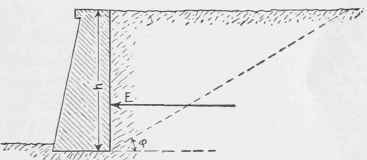In spite of the unreliability of theoretical formulae, for the reasons given above, certain formulæ which are here quoted without demonstration are sometimes used for lack of better formulæ as a guide in determining the thickness of a wall. For simplicity it is assumed that the rear face of the wall is vertical. The variation in the theory by attempting to allow for a slope of the rear face, merely complicates the theory; while the effect of such a variation from the vertical as is ever adopted is usually so small that it is utterly swallowed up by the unavoidable uncertainties in the practical application of the theory. In Fig. 66,Fig. 65. Typical Retaining Wall.

Let E = Total pressure against rear face of wall on a unit-length of wall; w = Weight of a unit-volume of the earth; h = Height of wall;

φ = The angle of repose with the horizontal - that is, the angle at [which that kind of earth will remain without further sliding.

Then, when the upper surface of the earth is horizontal, we have the equation:

E = tan2(45°-φ/2 ) wh2/2..(6)

If the upper surface of the earth is surcharged with a bank of earth at a natural slope, or if the angle of slope of the surcharge = φ, then the equation becomes:

E = 1/2 cos φ w h2..(7)

An inspection of Equation 6 will show that the pressure E is greater for small values of φ. The angle of repose for various materials is not only variable for different grades of material, but is variable for the same grade of material under various conditions of saturation. A value of φ which is frequently adopted is 30°.

This is considerably lower than the usual true value of φ tor dry material, and is usually a safe value of φ for any material (except quicksand) either wet or dry. The adoption of this value, therefore, generally means that the result is safe, and that the factor of safety is merely made somewhat larger.

225. Example

What will be the pressure per foot of length of the wall, for a wall 18 feet high, the angle of repose for the earth being assumed at 30°?

Solution. Here h = 18 feet, and φ = 30°. The weight of earth (w) is quoted as varying from 70 to 120 pounds per cubic foot according to the degree of saturation and density of packing. When the earth is densely packed, its angle of repose is greater; therefore we are safe in assuming a weight of 100 pounds per cubic foot for an angle (φ) of 30°. Substituting these values in Equation 6, we have:Fig. 66. Pressure on Retaining Wall.

E = tan2 (45o - 30o /2) 100 x 324

= tan2 30° X 16,200 = 5,400 pounds.

Using this value of φ = 30° gives us the simple relation that E = 1/6 w h2, or one-sixth of the unit-weight of the earth times the square of the height, for a wall without a surcharge.